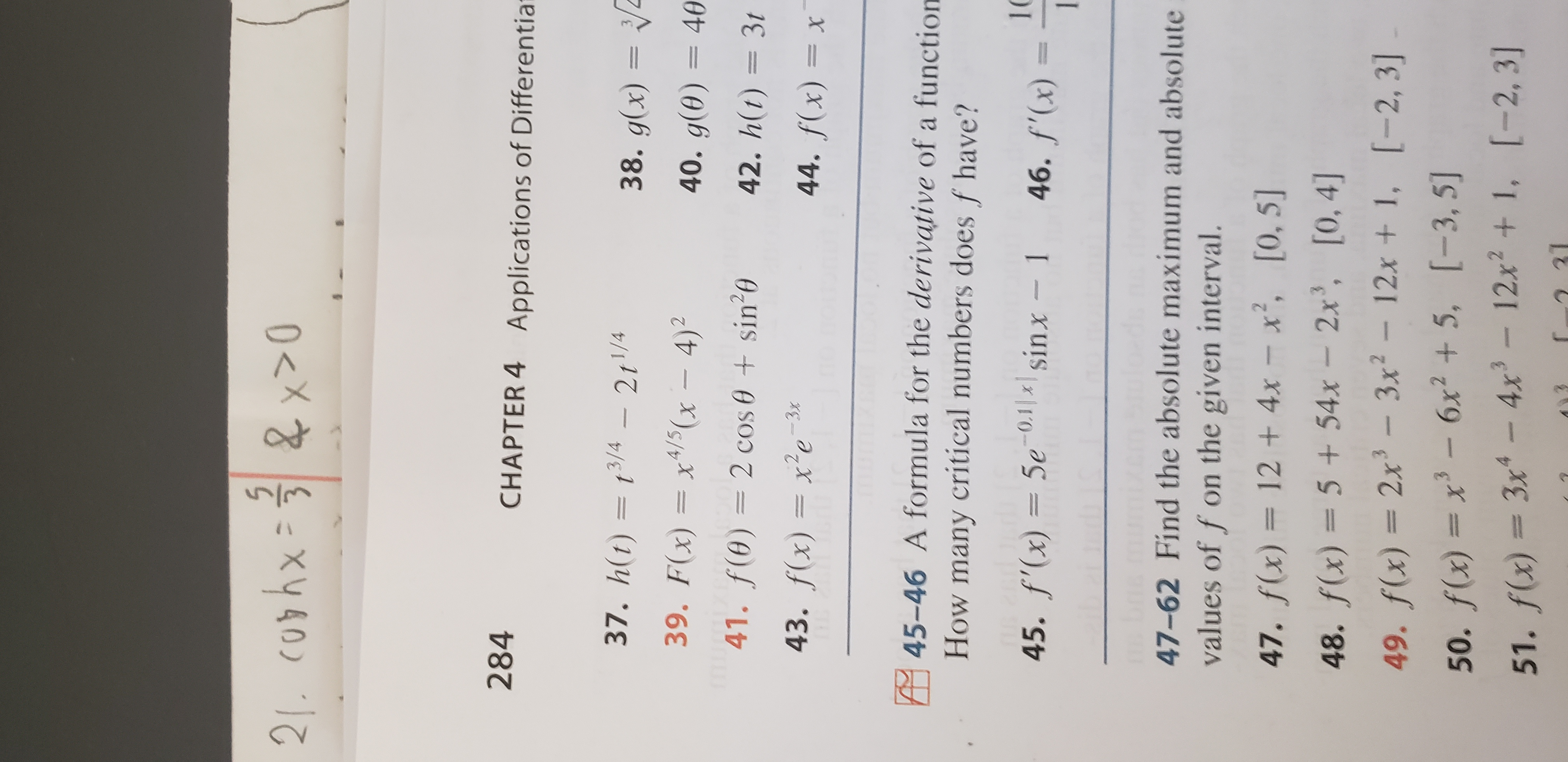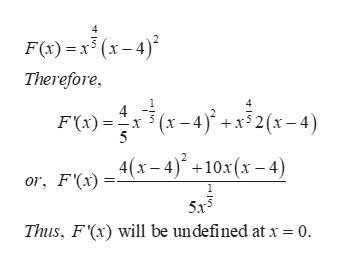# 21. c0phx x>0284CHAPTER 4Applications of Differentia37. h(t)= t3/4211/4- 2r1/438. g(x)= 439. F(x)= x4/5(x4)40. g(0)=4041. f(0)= 2 cos 0 sin 042. h(t) = 3t43. f(x)= xe244. f(x)= x45-46 A formula for the derivative of a functionHow many critical numbers does f have?1045. f'(x)= 5e0sinx-1 46. f'(x)147-62 Find the absolute maximum and absolutevalues of f on the given interval.12 + 4x - x, [0, 5]47. f(x)48. f(x) = 5 +54x - 2x3, [0, 4]49. f(x) 2x-3x2-12x + 1, [-2, 3]w50. f(x)= x- 6x2+ 5, [-3, 5]51. f(x) 3x-4x-12x2 + 1, [-2, 3131

Question
12 views

I need help with question 39 in Section 4.1, page 284, of the James Stewart Calculus Eighth Edition textbook. I am trying to find the critical numbers for the function provided.help_outlineImage Transcriptionclose21. c0phx x>0 284 CHAPTER 4 Applications of Differentia 37. h(t)= t3/4 211/4 - 2r1/4 38. g(x)= 4 39. F(x)= x4/5(x4) 40. g(0)=40 41. f(0)= 2 cos 0 sin 0 42. h(t) = 3t 43. f(x)= xe 2 44. f(x)= x 45-46 A formula for the derivative of a function How many critical numbers does f have? 10 45. f'(x)= 5e0sinx-1 46. f'(x) 1 47-62 Find the absolute maximum and absolute values of f on the given interval. 12 + 4x - x, [0, 5] 47. f(x) 48. f(x) = 5 +54x - 2x3, [0, 4] 49. f(x) 2x-3x2-12x + 1, [-2, 3] w 50. f(x)= x- 6x2+ 5, [-3, 5] 51. f(x) 3x-4x-12x2 + 1, [-2, 31 31 fullscreen
check_circle

Step 1

At first let us find the derivative of the function:help_outlineImage TranscriptioncloseF(x) x5 (x-4) Therefore 4 (x-4)+x32(x-4) F(x) 5 4(x-4)+10x (x-4) or, F(x) 5x3 Thus, F'(x) will be undefined at x = 0. 4 fullscreen
Step 2

Now, let us find where F'(x) is undefined. A...

### Want to see the full answer?

See Solution

#### Want to see this answer and more?

Solutions are written by subject experts who are available 24/7. Questions are typically answered within 1 hour.*

See Solution
*Response times may vary by subject and question.
Tagged in

### Derivative